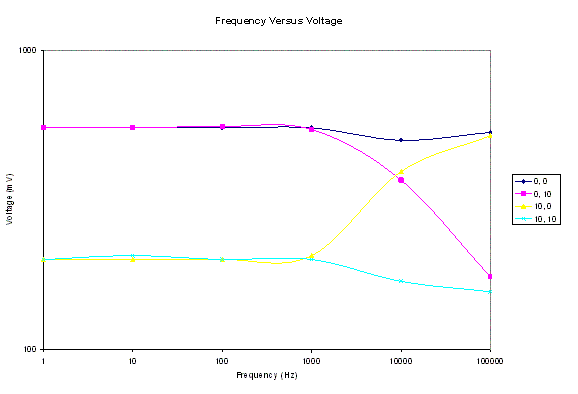Bill Chun Wai Hung

Peter Lau

Lab 11 Report �C Wrap up Lab

2 August 2004

A. Describe the Set-up

The Circuit setup is shown in figure 1 (without the line 2 input).Figure 1

B. Describe Inputs

Stimuli:

A range of input Frequency from 10Hz to 100kHz to see the output voltage response.

A range of AC input, from 500mV to 2V to observe the AC output to listen to the frequency.

Ra = 4.29k
R1 = R1��=510
C1=C2 = 47n
R2 = R2��= 510

Transformer: 115Vac to 12Vac transformer is used.

Diode: The model for the diodes are D1N4002

Voltage Regulator: The model for the Voltage Regulator is 7812, which gives an output voltage of 12V.

LED = Light Emitting Diode

C. Describe What you Observe

The output to the transfer function is not distorted by the changes made to this circuit. Therefore the output frequency response is the same as the frequency response recorded in lab7.

D. What you can deducePlot obtained from Lab07. Display here for convenience.

 Input Impedance 100k ohm (determined by the choice of  Rch1 or Rch2) Output Impedance 500 ohm (determined by the op amp used) Bandwidth The cutoff frequency for both the high pass and low pass filter is 5kHz. An valid input is the audio range. Maximum Attenuation According to the data from lab 7, maximum attenuation is 33%